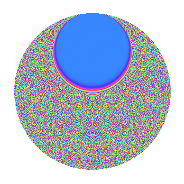Properties

 Label 4.34.a.aLevel 4 Weight 34 Character orbit 4.a Self dual Yes Analytic conductor 27.593 Analytic rank 0 Dimension 3 CM No Inner twists 1

Related objects

Newspace parameters

 Level: $$N$$ = $$4 = 2^{2}$$ Weight: $$k$$ = $$34$$ Character orbit: $$[\chi]$$ = 4.a (trivial)

Newform invariants

 Self dual: Yes Analytic conductor: $$27.5931315524$$ Analytic rank: $$0$$ Dimension: $$3$$ Coefficient field: $$\mathbb{Q}[x]/(x^{3} - \cdots)$$ Coefficient ring: $$\Z[a_1, \ldots, a_{5}]$$ Coefficient ring index: $$2^{22}\cdot 3^{3}\cdot 7\cdot 11\cdot 29$$ Fricke sign: $$-1$$ Sato-Tate group: $\mathrm{SU}(2)$

$q$-expansion

Coefficients of the $$q$$-expansion are expressed in terms of a basis $$1,\beta_1,\beta_2$$ for the coefficient ring described below. We also show the integral $$q$$-expansion of the trace form.

 $$f(q)$$ $$=$$ $$q$$ $$+ ( 30830596 + \beta_{1} ) q^{3}$$ $$+ ( -17960227962 - 1535 \beta_{1} + \beta_{2} ) q^{5}$$ $$+ ( 1513669971464 - 122346 \beta_{1} + 180 \beta_{2} ) q^{7}$$ $$+ ( 2010788144563341 + 75248670 \beta_{1} - 10530 \beta_{2} ) q^{9}$$ $$+O(q^{10})$$ $$q$$ $$+(30830596 + \beta_{1}) q^{3}$$ $$+(-17960227962 - 1535 \beta_{1} + \beta_{2}) q^{5}$$ $$+(1513669971464 - 122346 \beta_{1} + 180 \beta_{2}) q^{7}$$ $$+(2010788144563341 + 75248670 \beta_{1} - 10530 \beta_{2}) q^{9}$$ $$+(75872552434149900 + 2215839115 \beta_{1} + 256840 \beta_{2}) q^{11}$$ $$+(90990147405786254 + 6383188809 \beta_{1} - 3668535 \beta_{2}) q^{13}$$ $$+(-10714157827080368616 - 382917087630 \beta_{1} + 33406668 \beta_{2}) q^{15}$$ $$+(31012396103938905618 + 1069301430158 \beta_{1} - 184208050 \beta_{2}) q^{17}$$ $$+($$$$44\!\cdots\!76$$$$+ 11850406238205 \beta_{1} + 320516280 \beta_{2}) q^{19}$$ $$+(-$$$$76\!\cdots\!84$$$$- 57340224604180 \beta_{1} + 4392064620 \beta_{2}) q^{21}$$ $$+($$$$38\!\cdots\!32$$$$- 94999163347438 \beta_{1} - 49295676820 \beta_{2}) q^{23}$$ $$+($$$$86\!\cdots\!07$$$$+ 615284668657260 \beta_{1} + 277757168364 \beta_{2}) q^{25}$$ $$+($$$$38\!\cdots\!08$$$$+ 2919170542770738 \beta_{1} - 973938527640 \beta_{2}) q^{27}$$ $$+($$$$96\!\cdots\!26$$$$- 17291424904695515 \beta_{1} + 1814914414885 \beta_{2}) q^{29}$$ $$+($$$$52\!\cdots\!76$$$$+ 3377924000980200 \beta_{1} + 1180388959200 \beta_{2}) q^{31}$$ $$+($$$$17\!\cdots\!60$$$$+ 98072137402840890 \beta_{1} - 18904063453830 \beta_{2}) q^{33}$$ $$+($$$$34\!\cdots\!16$$$$+ 51799596297276380 \beta_{1} + 55139397898832 \beta_{2}) q^{35}$$ $$+($$$$11\!\cdots\!14$$$$- 1038005378158598451 \beta_{1} - 52265853646515 \beta_{2}) q^{37}$$ $$+($$$$45\!\cdots\!56$$$$+ 1463249000741125850 \beta_{1} - 130471960050900 \beta_{2}) q^{39}$$ $$+(-$$$$22\!\cdots\!54$$$$- 641632766910683060 \beta_{1} + 448924689742540 \beta_{2}) q^{41}$$ $$+(-$$$$51\!\cdots\!40$$$$+ 9557870528390708475 \beta_{1} - 210366843981840 \beta_{2}) q^{43}$$ $$+(-$$$$27\!\cdots\!62$$$$- 29103707165110673535 \beta_{1} - 950908515500799 \beta_{2}) q^{45}$$ $$+(-$$$$24\!\cdots\!92$$$$+ 13537930853481011508 \beta_{1} - 576631108849560 \beta_{2}) q^{47}$$ $$+(-$$$$15\!\cdots\!03$$$$+ 496168262546838840 \beta_{1} + 10601270675344440 \beta_{2}) q^{49}$$ $$+($$$$80\!\cdots\!12$$$$+$$$$13\!\cdots\!10$$$$\beta_{1} - 14436065202263640 \beta_{2}) q^{51}$$ $$+($$$$18\!\cdots\!78$$$$- 27337706038779017987 \beta_{1} - 40829066390340995 \beta_{2}) q^{53}$$ $$+($$$$24\!\cdots\!60$$$$-$$$$84\!\cdots\!50$$$$\beta_{1} + 158548384447532820 \beta_{2}) q^{55}$$ $$+($$$$92\!\cdots\!16$$$$+$$$$87\!\cdots\!06$$$$\beta_{1} - 119258077651337610 \beta_{2}) q^{57}$$ $$+($$$$67\!\cdots\!92$$$$+$$$$66\!\cdots\!35$$$$\beta_{1} - 355142845670518240 \beta_{2}) q^{59}$$ $$+($$$$19\!\cdots\!22$$$$+$$$$11\!\cdots\!45$$$$\beta_{1} + 864805591092629745 \beta_{2}) q^{61}$$ $$+(-$$$$41\!\cdots\!56$$$$-$$$$39\!\cdots\!06$$$$\beta_{1} - 321105448391693580 \beta_{2}) q^{63}$$ $$+(-$$$$75\!\cdots\!04$$$$-$$$$25\!\cdots\!20$$$$\beta_{1} - 991922296965526108 \beta_{2}) q^{65}$$ $$+(-$$$$96\!\cdots\!76$$$$+$$$$61\!\cdots\!09$$$$\beta_{1} + 745104731828763960 \beta_{2}) q^{67}$$ $$+(-$$$$50\!\cdots\!72$$$$+$$$$14\!\cdots\!80$$$$\beta_{1} + 150330017751397380 \beta_{2}) q^{69}$$ $$+($$$$56\!\cdots\!48$$$$+$$$$17\!\cdots\!70$$$$\beta_{1} + 4210979720475567620 \beta_{2}) q^{71}$$ $$+($$$$43\!\cdots\!34$$$$-$$$$54\!\cdots\!86$$$$\beta_{1} - 9892260507865032810 \beta_{2}) q^{73}$$ $$+($$$$67\!\cdots\!76$$$$+$$$$30\!\cdots\!55$$$$\beta_{1} - 1689547931514628848 \beta_{2}) q^{75}$$ $$+($$$$69\!\cdots\!40$$$$-$$$$13\!\cdots\!40$$$$\beta_{1} + 25628357491802757380 \beta_{2}) q^{77}$$ $$+($$$$14\!\cdots\!68$$$$+$$$$14\!\cdots\!60$$$$\beta_{1} - 24410580389385198840 \beta_{2}) q^{79}$$ $$+($$$$20\!\cdots\!09$$$$+$$$$38\!\cdots\!30$$$$\beta_{1} + 11004304993611004530 \beta_{2}) q^{81}$$ $$+(-$$$$23\!\cdots\!88$$$$-$$$$34\!\cdots\!63$$$$\beta_{1} - 30337551274260013440 \beta_{2}) q^{83}$$ $$+(-$$$$45\!\cdots\!28$$$$-$$$$41\!\cdots\!90$$$$\beta_{1} - 21434939812222425306 \beta_{2}) q^{85}$$ $$+(-$$$$84\!\cdots\!64$$$$-$$$$34\!\cdots\!14$$$$\beta_{1} +$$$$21\!\cdots\!80$$$$\beta_{2}) q^{87}$$ $$+(-$$$$64\!\cdots\!66$$$$+$$$$68\!\cdots\!30$$$$\beta_{1} -$$$$17\!\cdots\!70$$$$\beta_{2}) q^{89}$$ $$+(-$$$$12\!\cdots\!36$$$$-$$$$23\!\cdots\!40$$$$\beta_{1} -$$$$19\!\cdots\!40$$$$\beta_{2}) q^{91}$$ $$+($$$$18\!\cdots\!96$$$$+$$$$50\!\cdots\!76$$$$\beta_{1} - 15215953620938720400 \beta_{2}) q^{93}$$ $$+(-$$$$68\!\cdots\!92$$$$-$$$$46\!\cdots\!10$$$$\beta_{1} +$$$$56\!\cdots\!16$$$$\beta_{2}) q^{95}$$ $$+($$$$18\!\cdots\!74$$$$-$$$$10\!\cdots\!46$$$$\beta_{1} +$$$$87\!\cdots\!90$$$$\beta_{2}) q^{97}$$ $$+($$$$75\!\cdots\!00$$$$+$$$$14\!\cdots\!15$$$$\beta_{1} -$$$$27\!\cdots\!60$$$$\beta_{2}) q^{99}$$ $$+O(q^{100})$$ $$\operatorname{Tr}(f)(q)$$ $$=$$ $$3q$$ $$\mathstrut +\mathstrut 92491788q^{3}$$ $$\mathstrut -\mathstrut 53880683886q^{5}$$ $$\mathstrut +\mathstrut 4541009914392q^{7}$$ $$\mathstrut +\mathstrut 6032364433690023q^{9}$$ $$\mathstrut +\mathstrut O(q^{10})$$ $$3q$$ $$\mathstrut +\mathstrut 92491788q^{3}$$ $$\mathstrut -\mathstrut 53880683886q^{5}$$ $$\mathstrut +\mathstrut 4541009914392q^{7}$$ $$\mathstrut +\mathstrut 6032364433690023q^{9}$$ $$\mathstrut +\mathstrut 227617657302449700q^{11}$$ $$\mathstrut +\mathstrut 272970442217358762q^{13}$$ $$\mathstrut -\mathstrut 32142473481241105848q^{15}$$ $$\mathstrut +\mathstrut 93037188311816716854q^{17}$$ $$\mathstrut +\mathstrut$$$$13\!\cdots\!28$$$$q^{19}$$ $$\mathstrut -\mathstrut$$$$22\!\cdots\!52$$$$q^{21}$$ $$\mathstrut +\mathstrut$$$$11\!\cdots\!96$$$$q^{23}$$ $$\mathstrut +\mathstrut$$$$25\!\cdots\!21$$$$q^{25}$$ $$\mathstrut +\mathstrut$$$$11\!\cdots\!24$$$$q^{27}$$ $$\mathstrut +\mathstrut$$$$28\!\cdots\!78$$$$q^{29}$$ $$\mathstrut +\mathstrut$$$$15\!\cdots\!28$$$$q^{31}$$ $$\mathstrut +\mathstrut$$$$51\!\cdots\!80$$$$q^{33}$$ $$\mathstrut +\mathstrut$$$$10\!\cdots\!48$$$$q^{35}$$ $$\mathstrut +\mathstrut$$$$34\!\cdots\!42$$$$q^{37}$$ $$\mathstrut +\mathstrut$$$$13\!\cdots\!68$$$$q^{39}$$ $$\mathstrut -\mathstrut$$$$67\!\cdots\!62$$$$q^{41}$$ $$\mathstrut -\mathstrut$$$$15\!\cdots\!20$$$$q^{43}$$ $$\mathstrut -\mathstrut$$$$82\!\cdots\!86$$$$q^{45}$$ $$\mathstrut -\mathstrut$$$$72\!\cdots\!76$$$$q^{47}$$ $$\mathstrut -\mathstrut$$$$47\!\cdots\!09$$$$q^{49}$$ $$\mathstrut +\mathstrut$$$$24\!\cdots\!36$$$$q^{51}$$ $$\mathstrut +\mathstrut$$$$54\!\cdots\!34$$$$q^{53}$$ $$\mathstrut +\mathstrut$$$$72\!\cdots\!80$$$$q^{55}$$ $$\mathstrut +\mathstrut$$$$27\!\cdots\!48$$$$q^{57}$$ $$\mathstrut +\mathstrut$$$$20\!\cdots\!76$$$$q^{59}$$ $$\mathstrut +\mathstrut$$$$59\!\cdots\!66$$$$q^{61}$$ $$\mathstrut -\mathstrut$$$$12\!\cdots\!68$$$$q^{63}$$ $$\mathstrut -\mathstrut$$$$22\!\cdots\!12$$$$q^{65}$$ $$\mathstrut -\mathstrut$$$$29\!\cdots\!28$$$$q^{67}$$ $$\mathstrut -\mathstrut$$$$15\!\cdots\!16$$$$q^{69}$$ $$\mathstrut +\mathstrut$$$$16\!\cdots\!44$$$$q^{71}$$ $$\mathstrut +\mathstrut$$$$13\!\cdots\!02$$$$q^{73}$$ $$\mathstrut +\mathstrut$$$$20\!\cdots\!28$$$$q^{75}$$ $$\mathstrut +\mathstrut$$$$20\!\cdots\!20$$$$q^{77}$$ $$\mathstrut +\mathstrut$$$$43\!\cdots\!04$$$$q^{79}$$ $$\mathstrut +\mathstrut$$$$60\!\cdots\!27$$$$q^{81}$$ $$\mathstrut -\mathstrut$$$$71\!\cdots\!64$$$$q^{83}$$ $$\mathstrut -\mathstrut$$$$13\!\cdots\!84$$$$q^{85}$$ $$\mathstrut -\mathstrut$$$$25\!\cdots\!92$$$$q^{87}$$ $$\mathstrut -\mathstrut$$$$19\!\cdots\!98$$$$q^{89}$$ $$\mathstrut -\mathstrut$$$$38\!\cdots\!08$$$$q^{91}$$ $$\mathstrut +\mathstrut$$$$55\!\cdots\!88$$$$q^{93}$$ $$\mathstrut -\mathstrut$$$$20\!\cdots\!76$$$$q^{95}$$ $$\mathstrut +\mathstrut$$$$56\!\cdots\!22$$$$q^{97}$$ $$\mathstrut +\mathstrut$$$$22\!\cdots\!00$$$$q^{99}$$ $$\mathstrut +\mathstrut O(q^{100})$$

Basis of coefficient ring in terms of a root $$\nu$$ of $$x^{3}\mathstrut -\mathstrut$$ $$x^{2}\mathstrut -\mathstrut$$ $$65185566$$ $$x\mathstrut -\mathstrut$$ $$173679864984$$:

 $$\beta_{0}$$ $$=$$ $$1$$ $$\beta_{1}$$ $$=$$ $$($$$$-128 \nu^{2} + 542720 \nu + 5562320768$$$$)/25$$ $$\beta_{2}$$ $$=$$ $$($$$$343168 \nu^{2} + 6776698880 \nu - 14915325889408$$$$)/125$$
 $$1$$ $$=$$ $$\beta_0$$ $$\nu$$ $$=$$ $$($$$$5$$ $$\beta_{2}\mathstrut +\mathstrut$$ $$2681$$ $$\beta_{1}\mathstrut +\mathstrut$$ $$109756416$$$$)/$$$$329269248$$ $$\nu^{2}$$ $$=$$ $$($$$$1325$$ $$\beta_{2}\mathstrut -\mathstrut$$ $$3308935$$ $$\beta_{1}\mathstrut +\mathstrut$$ $$894316769246208$$$$)/20579328$$

Embeddings

For each embedding $$\iota_m$$ of the coefficient field, the values $$\iota_m(a_n)$$ are shown below.

For more information on an embedded modular form you can click on its label.

Label $$\iota_m(\nu)$$ $$a_{2}$$ $$a_{3}$$ $$a_{4}$$ $$a_{5}$$ $$a_{6}$$ $$a_{7}$$ $$a_{8}$$ $$a_{9}$$ $$a_{10}$$
1.1
 −6032.20 9172.25 −3139.05
0 −6.39317e7 0 −2.18954e11 0 −4.92542e13 0 −1.47179e15 0
1.2 0 2.16957e7 0 6.04966e11 0 1.12234e14 0 −5.08836e15 0
1.3 0 1.34728e8 0 −4.39893e11 0 −5.84388e13 0 1.25925e16 0
 $$n$$: e.g. 2-40 or 990-1000 Significant digits: Format: Complex embeddings Normalized embeddings Satake parameters Satake angles

Inner twists

This newform does not admit any (nontrivial) inner twists.

Atkin-Lehner signs

$$p$$ Sign
$$2$$ $$-1$$

Hecke kernels

There are no other newforms in $$S_{34}^{\mathrm{new}}(\Gamma_0(4))$$.